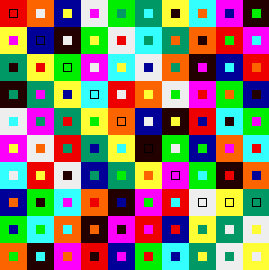## Graeco-Latin Squares

A Latin square of order n is a square array of size n that contains symbols from a set of size n. The symbols are arranged so that every row of the array has each symbol of the set occuring exactly once, and so that every column of the array has each symbol of the set also occuring exactly once.

Two Latin squares of order n are said to be orthogonal if one can be superimposed on the other, and each of the n^2 combinations of the symbols (taking the order of the superimposition into account) occurs exactly once in the n^2 cells of the array. Such pairs of orthogonal squares are often called Graeco-Latin squares since it is customary to use Latin letters for the symbols of one square and Greek letters for the symbols of the second square. In the example of a Graeco-Latin square of order 4 formed from playing cards, the two sets of symbols are the ranks (ace, king, queen and jack) and the suits (hearts, diamonds, clubs, spades). Here is an example of a Graeco-Latin square of order 10.An Order 10 Graeco-Latin Square (10K)

The two sets of "symbols" are identical - they are the 10 colors: red, purple, dark blue, light blue, light green, dark green, yellow, gray, black and brownish-orange. The larger squares constitute the Latin Square, while the inner squares constitute the Greek square. Every one of the 100 combination of colors (taking into account the distinction between the inner and outer squares) occurs exactly once. Note that for some elemnts of the array (principally, but not exclusively, along the diagonal) the inner and outer squares have the same color, rendering the distinction between them invisible.

Euler knew (c. 1780) that there was not a Graeco-Latin square of order 2 and knew constructions when n is odd or divisible by 4. Based on much experimentation, he conjectured that Graeco-Latin squares did not exist for orders of the form 4k + 2, k = 0, 1, 2, ... In 1901, Gaston Tarry proved (by exhaustive enumeration of the possible cases) that there was no Graeco-Latin square of order 6 - adding evidence to Euler's conjecture. However, in 1959, Parker, Bose and Shrikhande were able to construct an order 10 Graeco-Latin square, and provided a construction for the remaining even values of n that are not divisible by 4 (of course, excepting n = 2 and n = 6).

It can be proved that the size of the set of mutually pairwise orthogonal Latin squares of order n cannot exceed n - 1. The search for a set of 9 mutually pairwise orthogonal Latin squares of order 10 is equivalent to the search for a finite projective plane of order 10. A recent new result by Lam, Thiel and Swiercz (The non-existence of finite projective planes of order 10, Can. J. Math. XLI (1989) 1117-1123) used theoretical results to scale down a computer search to a reasonable size (approximately 2,000 hours on a CRAY). The resulting search led to the conclusion that a set of 9 mutually orthogonal Latin squares of order 10 does not exist.

To read an entertaining and informative account of Graeco-Latin Squares, consult "New Mathematical Diversions", by Martin Gardner, Simon & Schuster, 1966.

Rob Beezer, beezer@ups.edu, Updated: Jan 9, 1995, Created: Nov 9, 1994